Example Questions

Example Question #67 : Right Triangles

Given that two sides of a right triangle measure 2 feet and 3 feet, respectively, with a hypoteneuse of x, what is the perimeter of this right triangle (to the nearest tenth)?

3.6 feet

8.6 feet

18 feet

6.4 feet

9.4 feet

8.6 feet

Explanation:

Using the Pythagrean Theorem, we know that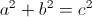.

This tells us: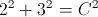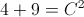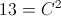Taking the square root of both sides, we find that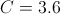To find the perimeter, we add the side lengths together, which gives us that the perimeter is: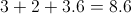Example Question #68 : Right Triangles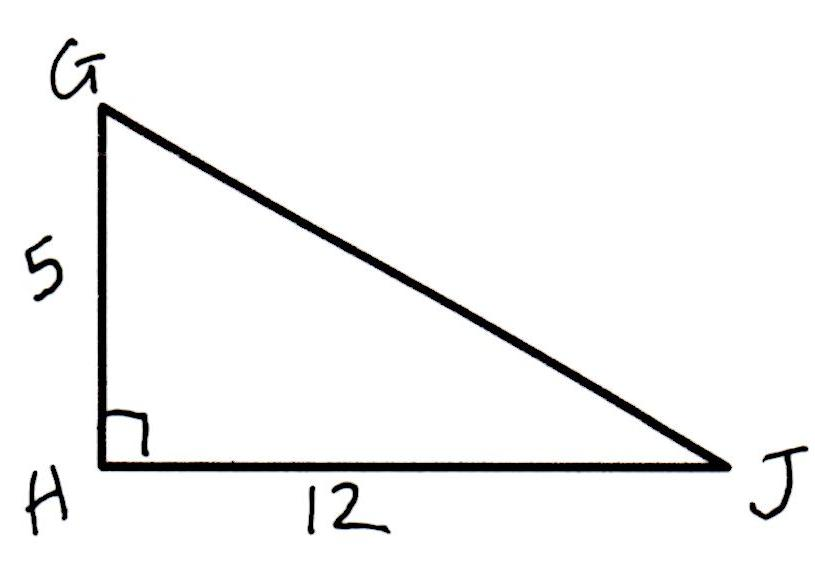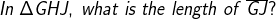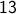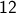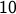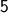Explanation: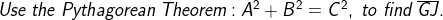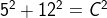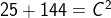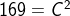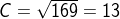Example Question #69 : Right Triangles

Kathy and Jill are travelling from their home to the same destination. Kathy travels due east and then after travelling 6 miles turns and travels 8 miles due north. Jill travels directly from her home to the destination. How miles does Jill travel?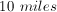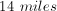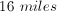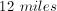Explanation:

Kathy's path traces the outline of a right triangle with legs of 6 and 8. By using the Pythagorean Theorem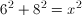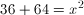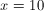miles

Example Question #70 : Right Triangles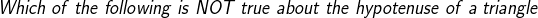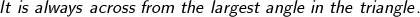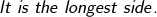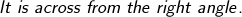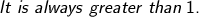Explanation: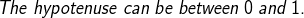Example Question #32 : How To Find The Length Of The Hypotenuse Of A Right Triangle : Pythagorean Theorem

In order to get to work, Jeff leaves home and drives 4 miles due north, then 3 miles due east, followed by 6 miles due north and, finally, 7 miles due east.  What is the straight line distance from Jeff’s work to his home?

2√5

11

6√2

15

10√2

10√2

Explanation:

Jeff drives a total of 10 miles north and 10 miles east.  Using the Pythagorean theorem (a2+b2=c2), the direct route from Jeff’s home to his work can be calculated.  102+102=c2.  200=c2. √200=c. √100√2=c. 10√2=c

Example Question #33 : How To Find The Length Of The Hypotenuse Of A Right Triangle : Pythagorean Theorem

Jim leaves his home and walks 10 minutes due west and 5 minutes due south. If Jim could walk a straight line from his current position back to his house, how far, in minutes, is Jim from home?

√5

6√6

5√5

√10

5√5

Explanation:

By using Pythagorean Theorem, we can solve for the distance “as the crow flies” from Jim to his home:

102 + 52 = x2

100 + 25 = x2

√125 = x, but we still need to factor the square root

√125 = √25*5, and since the √25 = 5, we can move that outside of the radical, so

5√5= x

Example Question #34 : How To Find The Length Of The Hypotenuse Of A Right Triangle : Pythagorean Theorem

A square enclosure has a total area of 3,600 square feet. What is the length, in feet, of a diagonal across the field rounded to the nearest whole number?

95

75

100

85

60

85

Explanation:

In order to find the length of the diagonal accross a square, we must first find the lengths of the individual sides.

The area of a square is found by multiply the lengths of 2 sides of a square by itself.

So, the square root of 3,600 comes out to 60 ft.

The diagonal of a square can be found by treating it like a right triangle, and so, we can use the pythagorean theorem for a right triangle.

602 + 602 = C2

the square root of 7,200 is 84.8, which can be rounded to 85

Example Question #35 : How To Find The Length Of The Hypotenuse Of A Right Triangle : Pythagorean Theorem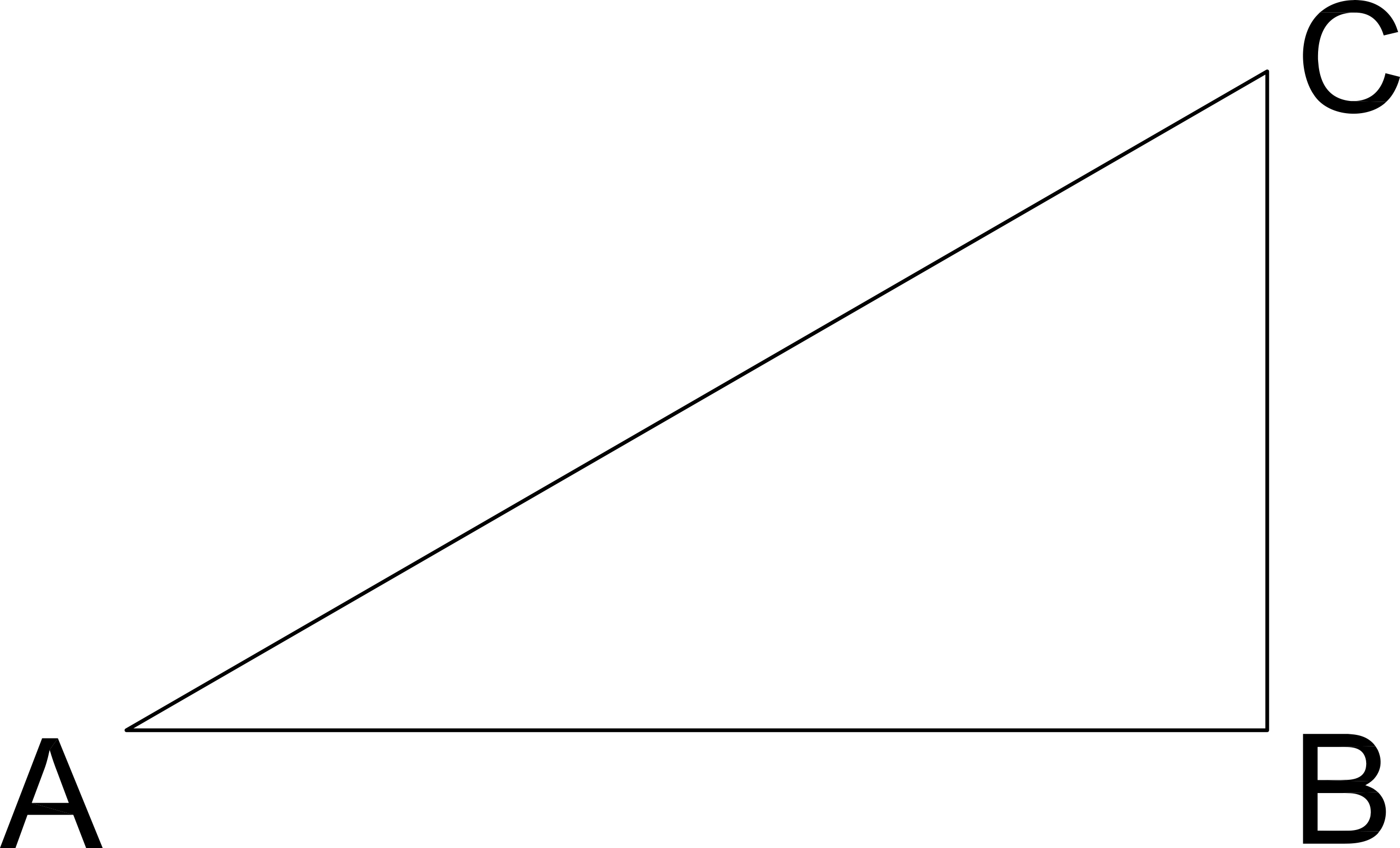If the length of CB is 6 and the angle C measures 45º, what is the length of AC in the given right triangle?

6

12√2

9

6√2

72

6√2

Explanation:

Pythagorean Theorum

AB2 + BC2 = AC2

If C is 45º then A is 45º, therefore AB = BC

AB2 + BC2 = AC2

62 + 62 = AC2

2*62 = AC2

AC = √(2*62) = 6√2

Example Question #36 : How To Find The Length Of The Hypotenuse Of A Right Triangle : Pythagorean Theorem

You leave on a road trip driving due North from Savannah, Georgia, at 8am.  You drive for 5 hours at 60mph and then head due East for 2 hours at 50mph.  After those 7 hours, how far are you Northeast from Savannah as the crow flies (in miles)?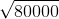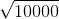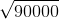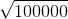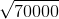Explanation:

Distance = hours * mph

North Distance = 5 hours * 60 mph = 300 miles

East Distance = 2 hours * 50 mph = 100 miles

Use Pythagorean Theorem to determine Northeast Distance

3002 + 1002 =NE2

90000  + 10000 = 100000 = NE2

NE = √100000

Example Question #37 : How To Find The Length Of The Hypotenuse Of A Right Triangle : Pythagorean Theorem

A square garden has an area of 49 ft2. To the nearest foot, what is the diagonal distance across the garden?

8

10

11

7

9